Want to share your content on R-bloggers? click here if you have a blog, or here if you don't.

This September we went on a roadtrip to the US and Canada. Of course, we had our trusty GPS to guide us along the way – the data from which I downloaded and used to play around with.

(If you want to see a few photos from the trip and don’t care about the rest, skip to the bottom…)

I used the XML package to load the gpx files following this blog post.

```library(XML)

myfiles <- list.files(path = "../gpx/gpx_files", full.names = TRUE)

for (i in 1:length(myfiles)){

# One of the files seems to be broken, but since the file is one with earlier data
# not from the trip, I will simply skip it
tryCatch({
pfile <- htmlTreeParse(myfiles[i], useInternalNodes = T)

}, error=function(e){cat("ERROR\n")})

if (exists("pfile")){
# Get all elevations, times and coordinates via the respective xpath
elevations <- as.numeric(as.character(xpathSApply(pfile, path = "//trkpt/ele", xmlValue)))
times <- xpathSApply(pfile, path = "//trkpt/time", xmlValue)
coords <- xpathSApply(pfile, path = "//trkpt", xmlAttrs)
# Extract latitude and longitude from the coordinates
lats <- as.numeric(as.character(coords["lat",]))
lons <- as.numeric(as.character(coords["lon",]))
# Put everything in a dataframe and get rid of old variables
geodf <- data.frame(lat = lats, lon = lons, ele = elevations, time = times)

if (i == 1){
geodata <- geodf
} else {
geodata <- rbind(geodata, geodf)
}
}
rm(pfile)
}
geodata_all <- geodata
```

I then did some cleaning of the date and time column and removed all the old track that were not part of our trip.

```# Transforming the time column
geodata_all\$time <- strptime(geodata_all\$time, format = "%Y-%m-%dT%H:%M:%SZ")

# ordering by date
library(plyr)
geodata_all <- arrange(geodata_all, time)

# removing all tracks from Germany
geodata <- geodata_all[which(as.numeric(as.character(geodata_all\$lon)) < 0),]

# adding a column with day for separating the plotting by day lateron
geodata\$day <- as.factor(format(as.Date(geodata\$time,format="%Y-%m-%d"), "%d"))
```

We have 119225 data points with a range from 2016-09-09 19:23:53 to 2016-09-23 21:36:06.

```summary(geodata)
```
```##       lat             lon              ele
##  Min.   :36.99   Min.   :-88.68   Min.   :-26.78
##  1st Qu.:40.09   1st Qu.:-84.86   1st Qu.:181.83
##  Median :41.90   Median :-82.99   Median :198.65
##  Mean   :41.27   Mean   :-82.76   Mean   :216.58
##  3rd Qu.:42.33   3rd Qu.:-80.44   3rd Qu.:255.85
##  Max.   :43.65   Max.   :-78.85   Max.   :438.02
##
##       time                          day
##  Min.   :2016-09-09 19:23:53   19     :17203
##  1st Qu.:2016-09-12 12:14:47   12     :14455
##  Median :2016-09-15 21:52:37   11     :14020
##  Mean   :2016-09-15 22:35:18   10     :12061
##  3rd Qu.:2016-09-19 15:59:09   16     :10856
##  Max.   :2016-09-23 21:36:06   13     :10787
##                                (Other):39843
```
```str(geodata)
```
```## 'data.frame':    119225 obs. of  5 variables:
##  \$ lat : num  42 42 42 42 42 ...
##  \$ lon : num  -87.9 -87.9 -87.9 -87.9 -87.9 ...
##  \$ ele : num  122 122 122 122 122 ...
##  \$ time: POSIXlt, format: "2016-09-09 19:23:53" "2016-09-09 19:23:53" ...
##  \$ day : Factor w/ 15 levels "09","10","11",..: 1 1 1 1 1 1 1 1 1 1 ...
```

Somehow the GPS didn’t record the last two days of our trip back to Chicago, so I added a few way points with the Google Maps Geocoding and Directions APIs (accessed via the googleway package).

```library(googleway)

# on th 26th we had lunch at cracker barrel
cracker_barrel <- google_geocode(address = "2101 N Kenyon Rd, Urbana, IL 61802, USA",
key = "your_key",
language = "en",
simplify = TRUE)

cracker_barrel_data <- data.frame(lat = cracker_barrel\$results\$geometry\$location\$lat,
lon = cracker_barrel\$results\$geometry\$location\$lng,
ele = "NA", time = as.POSIXlt("2016-09-26 19:00:00"))

# then we stayed the night in Aurora/ Naperville
key = "your_key",
language = "en",
simplify = TRUE)

super8_data <- data.frame(lat = super8\$results\$geometry\$location\$lat,
lon = super8\$results\$geometry\$location\$lng,
ele = "NA", time = as.POSIXlt("2016-09-26 23:30:00"))

# on the 27th we went back to the airport to fly home
ohare <- google_geocode(address = "10000 W O'Hare Ave, Chicago, IL 60666, USA",
key = "your_key",
language = "en",
simplify = TRUE)

ohare_data <- data.frame(lat = ohare\$results\$geometry\$location\$lat,
lon = ohare\$results\$geometry\$location\$lng,
ele = "NA", time = as.POSIXlt("2016-09-27 18:00:00"))
```

Using only these three waypoints won’t plot nicely on a map because it will draw straight lines between the points. So, I also want to get travel information from Google Maps between these points to get a few more waypoints along the actual route we drove.

```## Route from Paducah to Cracker Barrel

destination = c(cracker_barrel_data\$lat, cracker_barrel_data\$lon),
mode = "driving",
key = "your_key",
language = "en",
simplify = TRUE)

## Route from Cracker Barrel to Aurora

route_cracker_aurora <- google_directions(origin = c(cracker_barrel_data\$lat, cracker_barrel_data\$lon),
destination = c(super8_data\$lat, super8_data\$lon),
mode = "driving",
key = "your_key",
language = "en",
simplify = TRUE)

route_cracker_aurora_legs <- as.data.frame(route_cracker_aurora\$routes\$legs)
route_cracker_aurora_legs_steps <- as.data.frame(route_cracker_aurora_legs\$steps)

## Route from Cracker Aurora to O'Hare

route_aurora_ohare <- google_directions(origin = c(super8_data\$lat, super8_data\$lon),
destination = c(ohare_data\$lat, ohare_data\$lon),
mode = "driving",
key = "your_key",
language = "en",
simplify = TRUE)

route_aurora_ohare_legs <- as.data.frame(route_aurora_ohare\$routes\$legs)
route_aurora_ohare_legs_steps <- as.data.frame(route_aurora_ohare_legs\$steps)

# Combining the data sets
geodata_2 <- data.frame(lat = c(geodata\$lat,
route_cracker_aurora_legs\$start_location\$lat, route_cracker_aurora_legs_steps\$start_location\$lat,
route_cracker_aurora_legs\$end_location\$lat, super8_data\$lat,
route_aurora_ohare_legs\$start_location\$lat, route_aurora_ohare_legs_steps\$start_location\$lat,
route_aurora_ohare_legs\$end_location\$lat, ohare_data\$lat),
route_cracker_aurora_legs\$start_location\$lng, route_cracker_aurora_legs_steps\$start_location\$lng,
route_cracker_aurora_legs\$end_location\$lng, super8_data\$lon,
route_aurora_ohare_legs\$start_location\$lng, route_aurora_ohare_legs_steps\$start_location\$lng,
route_aurora_ohare_legs\$end_location\$lng, ohare_data\$lon),
day = c(as.character(geodata\$day), rep("26",
route_cracker_aurora_legs\$start_location\$lat,
route_cracker_aurora_legs_steps\$start_location\$lat,
route_cracker_aurora_legs\$end_location\$lat, super8_data\$lat))),
rep("27",
length(c(route_aurora_ohare_legs\$start_location\$lat, route_aurora_ohare_legs_steps\$start_location\$lat,
route_aurora_ohare_legs\$end_location\$lat, ohare_data\$lat)))))
```

## Plotting

I tried several packages for plotting but ended up with ggmap as the nicest looking solution.

### Overview of the trip

We flew to Chicago, went west across Michigan to Ann Arbor, Detroit, Toronto, then south again to Pittsburgh, from where we followed the Ohio river and headed to Louisville. From there, we went to Paducah, KY and back north again to Chicago. Each color shows a different day.

```library(ggmap)

bc_bbox <- make_bbox(lat = lat, lon = lon, data = geodata_2, f = .10)
map <- get_map(location = bc_bbox, maptype = "roadmap")

ggmap(map, darken = c(0.3, "white")) + geom_point(aes(x = lon, y = lat, col = factor(day), group = factor(day)), data = geodata_2) +
geom_path(aes(x = lon, y = lat, col = factor(day)), data = geodata_2[which(geodata_2\$day %in% c("26", "27")), ]) +
theme(legend.position="none") + labs(x="Longitude", y="Latitude", title="Roadtrip 2016")
```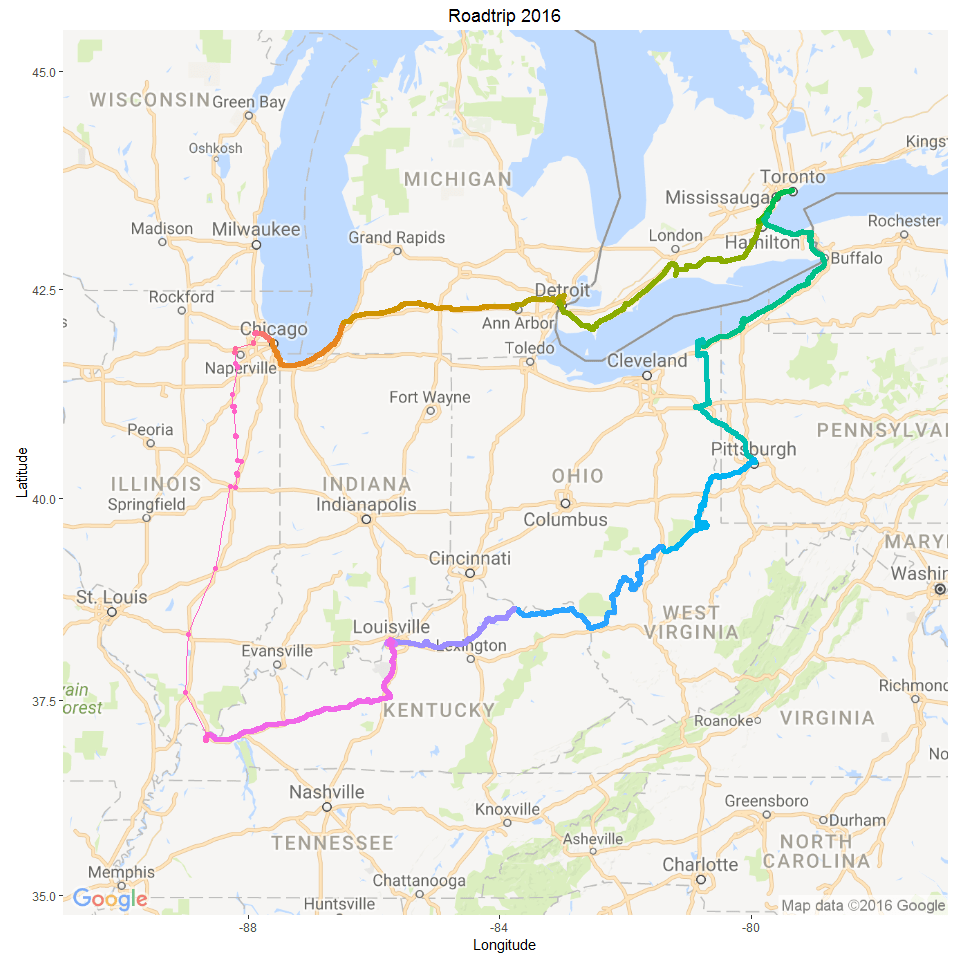First, I need to obtain the geocodes from the stops (again via Google Maps API).

```chicago <- google_geocode(address = "Chicago",
key = "your_key",
language = "en",
simplify = TRUE)

key = "your_key",
language = "en",
simplify = TRUE)

key = "your_key",
language = "en",
simplify = TRUE)

key = "your_key",
language = "en",
simplify = TRUE)

key = "your_key",
language = "en",
simplify = TRUE)

key = "your_key",
language = "en",
simplify = TRUE)

key = "your_key",
language = "en",
simplify = TRUE)

key = "your_key",
language = "en",
simplify = TRUE)

key = "your_key",
language = "en",
simplify = TRUE)

key = "your_key",
language = "en",
simplify = TRUE)

key = "your_key",
language = "en",
simplify = TRUE)

ann_text <- data.frame(x = c(chicago\$results\$geometry\$location\$lng,
stjospeh\$results\$geometry\$location\$lng,
annarbor\$results\$geometry\$location\$lng,
detroit\$results\$geometry\$location\$lng,
burlington\$results\$geometry\$location\$lng,
ashtabula\$results\$geometry\$location\$lng,
pittsburgh\$results\$geometry\$location\$lng,
marietta\$results\$geometry\$location\$lng,
maysville\$results\$geometry\$location\$lng,
louisville\$results\$geometry\$location\$lng, #Maps found a second entry for the location
y = c(chicago\$results\$geometry\$location\$lat,
stjospeh\$results\$geometry\$location\$lat,
annarbor\$results\$geometry\$location\$lat,
detroit\$results\$geometry\$location\$lat+0.1, #adjusting Detroit label to avoid overlapping with Ann Arbor
burlington\$results\$geometry\$location\$lat,
ashtabula\$results\$geometry\$location\$lat,
pittsburgh\$results\$geometry\$location\$lat,
marietta\$results\$geometry\$location\$lat,
maysville\$results\$geometry\$location\$lat,
louisville\$results\$geometry\$location\$lat,
))
```
```map <- get_map(location = bc_bbox, maptype = "watercolor")

roadtrip_map <- ggmap(map, darken = c(0.3, "white")) + geom_point(aes(x = lon, y = lat, col = factor(day), group = factor(day)), data = geodata_2, alpha = 0.3) +
geom_path(aes(x = lon, y = lat, col = factor(day)), data = geodata_2[which(geodata_2\$day %in% c("26", "27")), ], alpha = 0.5) +
theme(legend.position="none") + labs(x="Longitude", y="Latitude", title="Roadtrip 2016")
roadtrip_map + geom_text(data = ann_text, aes(x, y, label = labs), size = 4)
```#### How many kilometers did we drive?

```# removing duplicate entries
geodata_2 <- geodata_2[!duplicated(geodata_2), ]

# Shifting vectors for latitude and longitude to include end position
shift.vec <- function (vec, shift){
if(length(vec) <= abs(shift)){
rep(NA ,length(vec))
} else {
if (shift >= 0) {
c(rep(NA, shift), vec[1:(length(vec)-shift)]) }
else {
c(vec[(abs(shift)+1):length(vec)], rep(NA, abs(shift)))
}
}
}

geodata_2\$lat.p1 <- shift.vec(geodata_2\$lat, -1)
geodata_2\$lon.p1 <- shift.vec(geodata_2\$lon, -1)

# Calculating distances between points (in metres) with the function pointDistance from the 'raster' package.

library(raster)
geodata_2\$dist.to.prev <- apply(geodata_2, 1, FUN = function (row) {
pointDistance(c(as.numeric(as.character(row["lat.p1"])),
as.numeric(as.character(row["lon.p1"]))),
c(as.numeric(as.character(row["lat"])), as.numeric(as.character(row["lon"]))),
lonlat = T) # Parameter 'lonlat' has to be TRUE!
})
```

We drove approximately 3236.95 km in 18 days. Not counting the ~6 days where we stayed in one place and didn’t drive much, that’s around 269.75 km/ day.

### Identifying local time

The time stamps are in CEST - Central European Summer Time. We arrived in the Central Time Zone (CDT) but spent the majority of our trip in Estern Time Zone (EDT).

#### Converting to CDT and EDT

```library(lubridate)

geodata\$time_CDT <- with_tz(geodata\$time, tz="America/Chicago")
geodata\$time_EDT <- with_tz(geodata\$time, tz="America/New_York")

# extracting day for EDT
geodata\$day_EDT <- as.factor(format(as.Date(geodata\$time_EDT,format="%Y-%m-%d"), "%d"))
```

#### Identifying the local time based on geocode

```# the days where we crossed time zones were the 10th and 23rd

geodata_timezone <- geodata
geodata_timezone\$local_time <- "NA"

for (i in 1:nrow(geodata)){
if (is.na(nchar(strsplit(as.character(i/100), "\\.")[]))){
print(i)
}

if (geodata[i, "day_EDT"] == "10"){
data_row <- geodata[i, ]
timestamp = as.POSIXct(data_row\$time_EDT), # because the majority of our trip was in EDT
key = "AIzaSyBkqrk8mBqqvao-W-jKkL2VhHCGLWqZVCY",
simplify = TRUE,
language = "en")

local_time <- as.POSIXlt(with_tz(data_row\$time, tz=paste(time_zone)))

geodata_timezone\$local_time[i] <- as.character(local_time)

} else {

if (geodata[i, "day_EDT"] == "09"){
geodata_timezone\$local_time[i] <- as.character(geodata_timezone\$time_CDT[i])
} else {
geodata_timezone\$local_time[i] <- as.character(geodata_timezone\$time_EDT[i])
}
}
}
```

### Plotting elevation

```theme_set(theme_bw()) # Change the theme to my preference

labels <- c("9" = "9th, Chicago", "10" = "10th, St. Joseph", "11" = "11th, Ann Arbor", "12" = "12th, Detroit", "13" = "13th, Burlington",
"14" = "14th, Burlington", "15" = "15th, Toronto", "16" = "16th, Niagara & Ashtabula", "17" = "17th, Pittsburgh",
"18" = "18th, Pittsburgh", "19" = "19th, Marietta", "20" = "20th, Maysville", "21" = "21st, Louisville",
"22" = "22nd, Louisville", "23" = "23rd, Paducah")

ggplot(aes(x = as.POSIXlt(local_time), y = ele), data = geodata_timezone) +
geom_point(size = 0.5) + facet_wrap( ~ day_EDT, ncol=3, scales = "free_x", labeller=labeller(day_EDT = labels)) +
labs(x="Local Time", y="Elevation", title="Elevation per day and time")
```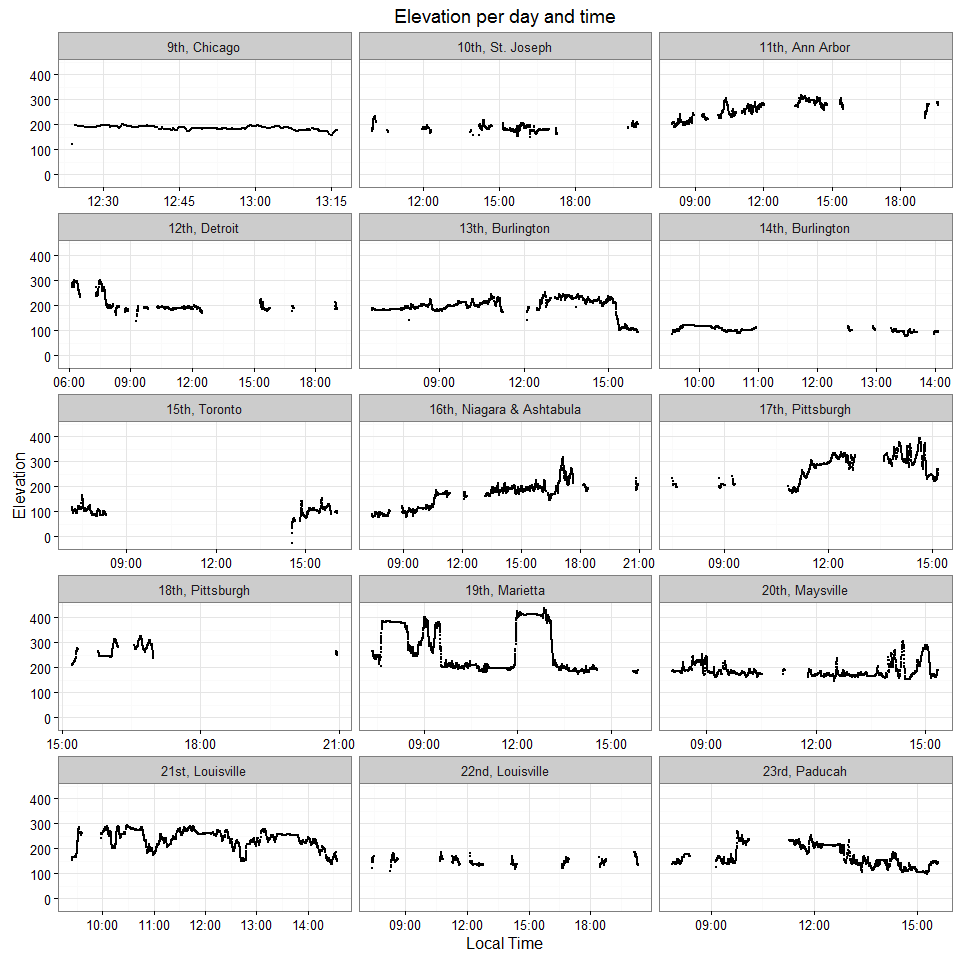```day_df <- data.frame(row.names = unique(geodata_timezone\$day_EDT),
group = unique(geodata_timezone\$day_EDT),
xmin = rep(NA, length(unique(geodata_timezone\$day_EDT))),
xmax = rep(NA, length(unique(geodata_timezone\$day_EDT))),
ymin = rep(-Inf, length(unique(geodata_timezone\$day_EDT))),
ymax = rep(Inf, length(unique(geodata_timezone\$day_EDT))))

for (day in unique(geodata_timezone\$day_EDT)){
if (!day == "23"){
day_df[paste(day), 2] <- which(geodata_timezone\$day_EDT == day)
day_df[paste(day), 3] <- which(geodata_timezone\$day_EDT == as.numeric(as.character(day))+1)-1
} else {
day_df[paste(day), 2] <- which(geodata_timezone\$day_EDT == day)
day_df[paste(day), 3] <- nrow(geodata_timezone)
}
}

ggplot(aes(x = seq_along(ele), y = ele), data = geodata_timezone) +
geom_rect(data=day_df, inherit.aes=FALSE,
aes(xmin=xmin, xmax=xmax, ymin=ymin, ymax=ymax, fill = factor(group)), col = "grey", alpha=0.5) +
#annotate("rect", xmin=0, xmax=0, ymin=-Inf, ymax=0, fill="red", alpha=0.5) +
geom_path() +
labs(x="Index", y="Elevation (line) and day (rectangles)", title="Elevation per day") +
scale_y_continuous(limits = c(min(c(geodata_timezone\$ele, as.numeric(as.character(geodata_timezone\$day_EDT)))),
max(c(geodata_timezone\$ele, as.numeric(as.character(geodata_timezone\$day_EDT)))))) +
scale_fill_discrete(name="Days", labels = labels)
```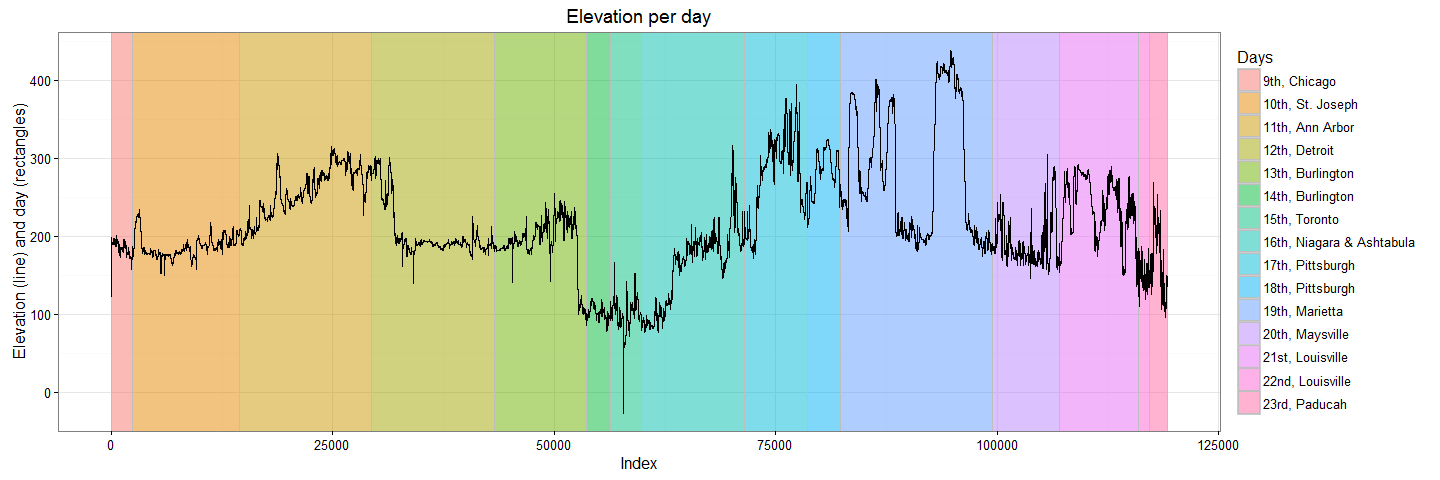## Extracting speed data from the gpx files

Looking at the gpx file, there is also speed and course data. It is saved into the extensions tag. However, not all data points seem to have a speed tag.

```for (i in 1:length(myfiles)){

tryCatch({
pfile2 <- gsub("</time><extensions><gpxtpx:TrackPointExtension><gpxtpx:course>",
"</time><extensions><gpxtpx:TrackPointExtension><gpxtpx:speed>0.00</gpxtpx:speed><gpxtpx:course>", singleString)

pfile <- htmlTreeParse(pfile2, useInternalNodes = T)

# Get all elevations, times and coordinates via the respective xpath
times <- xpathSApply(pfile, path = "//trkpt/time", xmlValue)
coords <- xpathSApply(pfile, path = "//trkpt", xmlAttrs)
# Extract latitude and longitude from the coordinates
lats <- as.numeric(as.character(coords["lat",]))
lons <- as.numeric(as.character(coords["lon",]))

speed <- xpathSApply(pfile, path = "//trkpt/extensions/*/speed", xmlValue)# in m/s

# course gives the degrees where the car was headed at that point
course <- xpathSApply(pfile, path = "//trkpt/extensions/*/course", xmlValue)
# Put everything in a dataframe and get rid of old variables
geodf <- data.frame(lat = lats, lon = lons, time = times, speed = speed, course = course)

if (i == 1){
geodata_new <- geodf
} else {
geodata_new <- rbind(geodata_new, geodf)
}

rm(pfile, pfile2, singleString)
}, error=function(e){cat("ERROR\n")})

}
```
```# Transforming the time column
geodata_new\$time <- strptime(geodata_new\$time, format = "%Y-%m-%dT%H:%M:%SZ")

# ordering by date
geodata_new <- arrange(geodata_new, time)

# removing all tracks from Germany
geodata_new_trip <- geodata_new[which(as.numeric(as.character(geodata_new\$lon)) < 0),]

# adding a column with day for separating the plotting by day lateron
geodata_new_trip\$day <- as.factor(format(as.Date(geodata_new_trip\$time,format="%Y-%m-%d"), "%d"))

```
```##            lat      lon                time speed course day
## 35664 41.98135 -87.8818 2016-09-09 19:23:53  0.00   0.00  09
## 35665 41.98135 -87.8818 2016-09-09 19:23:53  0.00   0.00  09
## 35666 41.98135 -87.8818 2016-09-09 19:23:53  0.00   0.00  09
## 35667 41.98135 -87.8818 2016-09-09 19:23:53  0.00   0.00  09
## 35668 41.98135 -87.8818 2016-09-09 19:23:53  0.00   0.00  09
## 35669 41.98135 -87.8818 2016-09-09 19:23:53  0.00   0.00  09
```

Garmin shows speed as m/s, so I am converting it to km/h.

```library(yarrr)

geodata_new_trip\$speed_kmh <- as.numeric(as.character(geodata_new_trip\$speed)) *3.6

speed_data <- geodata_new_trip[which(as.numeric(as.character(geodata_new_trip\$speed_kmh)) > 0),]
speed_data <- speed_data[!duplicated(speed_data), ]

summary(as.numeric(as.character(speed_data\$speed_kmh)))
```
```##    Min. 1st Qu.  Median    Mean 3rd Qu.    Max.
##   4.932  24.700  44.460  49.210  69.190 128.500
```
```pirateplot(formula = as.numeric(as.character(speed_kmh)) ~ as.factor(day),
point.o = .1,
data = speed_data,
ylab = "Speed (km/h)",
xlab = "Day in September",
main = "Speed per day")
```## Photos

And finally some photos from our trip. Their locations are marked on the map below:

```myphotos <- list.files(path = ".", full.names = TRUE)
myphotos <- myphotos[grep("jpg", myphotos)]

library(exif)

photo_geotags <- data.frame(row.names = gsub("./", "", myphotos), lat = rep(NA, length(myphotos)), lon = NA)

for (file in gsub("./", "", myphotos)){
lat <- photodata\$latitude
lon <- photodata\$longitude
photo_geotags[file, ]\$lat <- lat
photo_geotags[file, ]\$lon <- lon
}

photo_geotags\$text <- seq(1:nrow(photo_geotags))

library(ggmap)
library(ggrepel)

bc_bbox <- make_bbox(lat = lat, lon = lon, data = photo_geotags, f = .10)
map <- get_map(location = bc_bbox, maptype = "roadmap")

ggmap(map, darken = c(0.3, "white")) +
geom_point(aes(x = lon, y = lat), data = photo_geotags) +
theme(legend.position="none") + labs(x="Longitude", y="Latitude", title="Roadtrip 2016") +
geom_text_repel(aes(label = text), data = photo_geotags)
```Photo number 1Photo number 2Photo number 3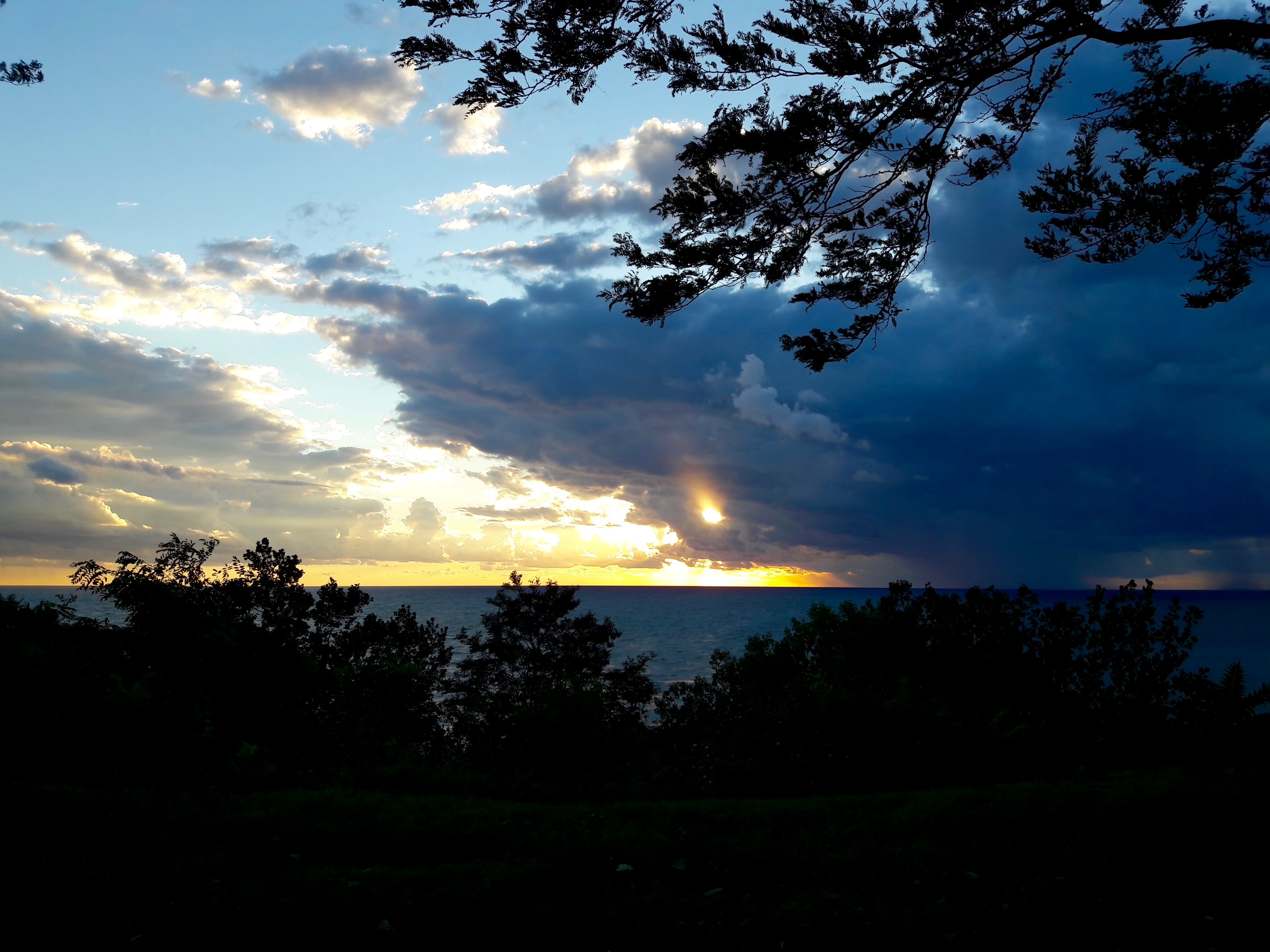Photo number 4Photo number 5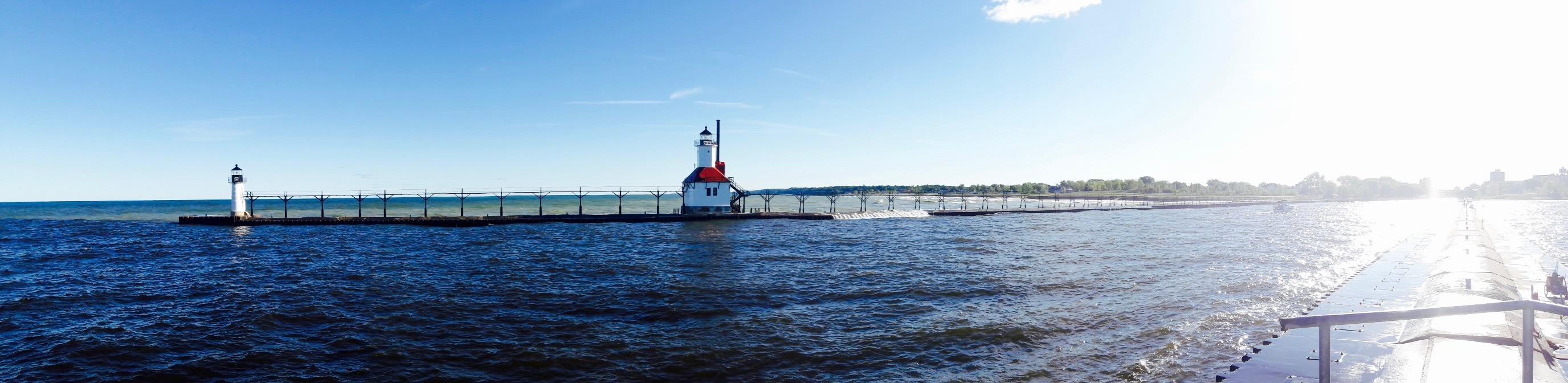Photo number 6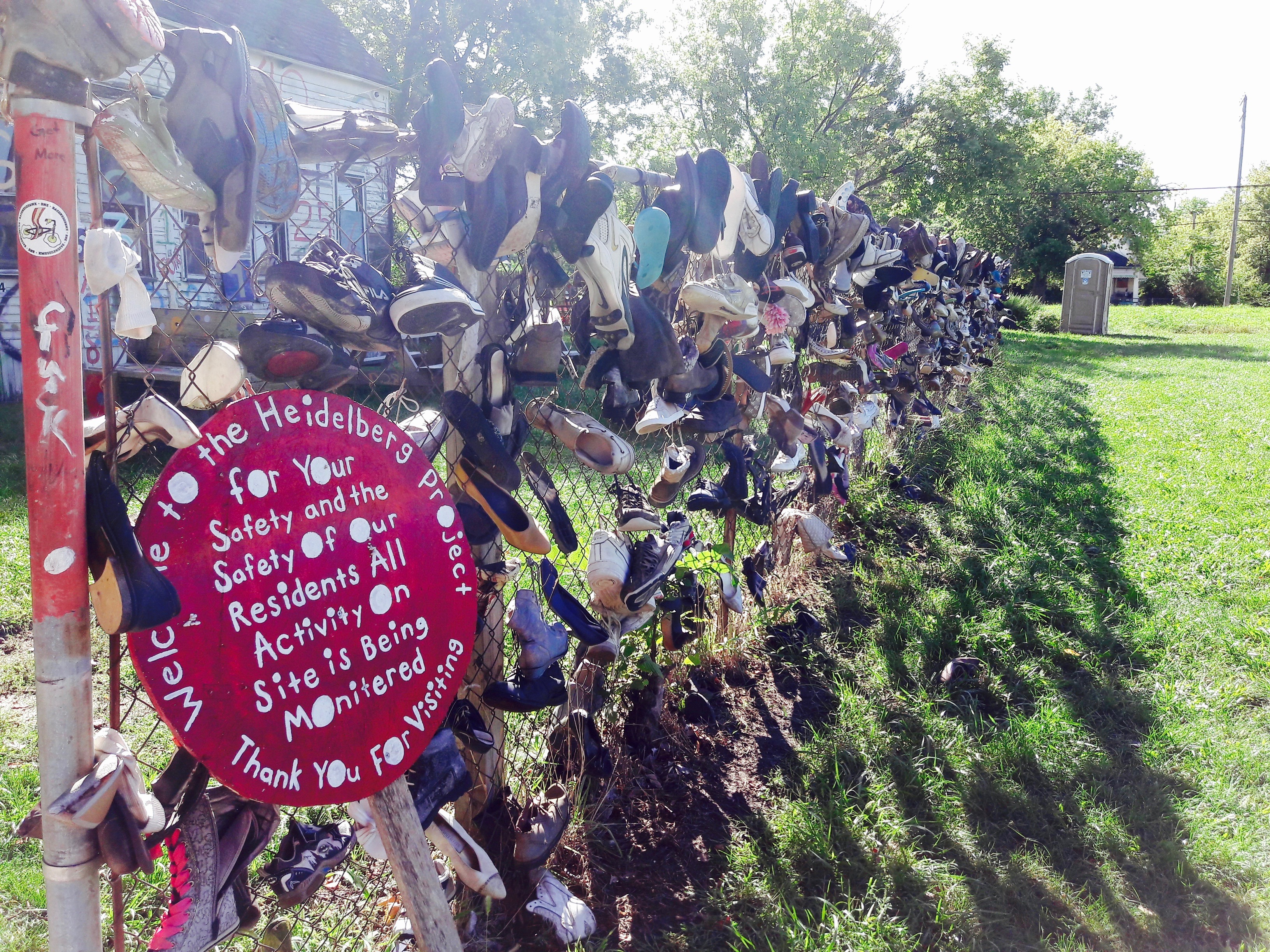Photo number 7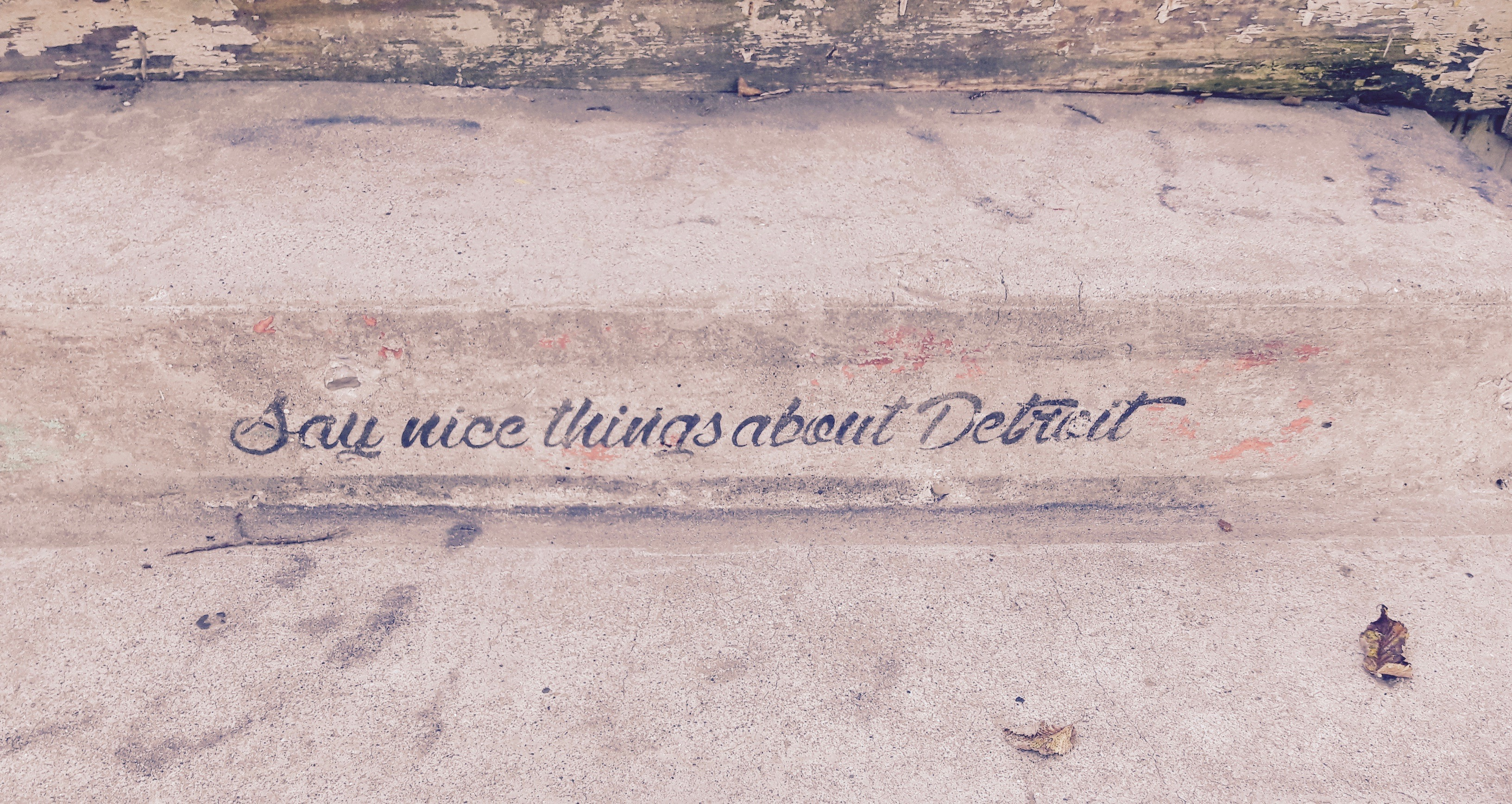Photo number 8Photo number 9Photo number 10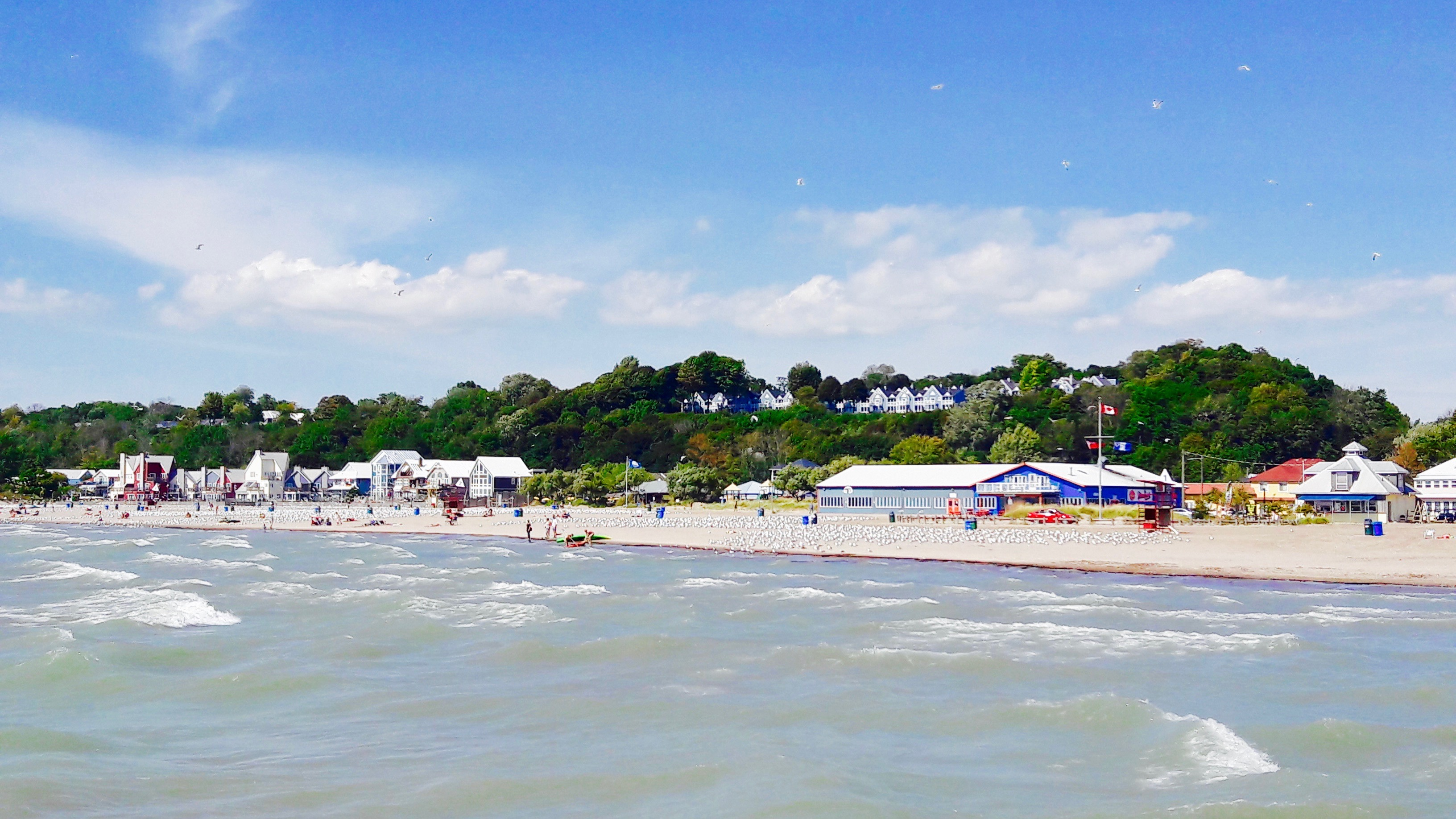Photo number 11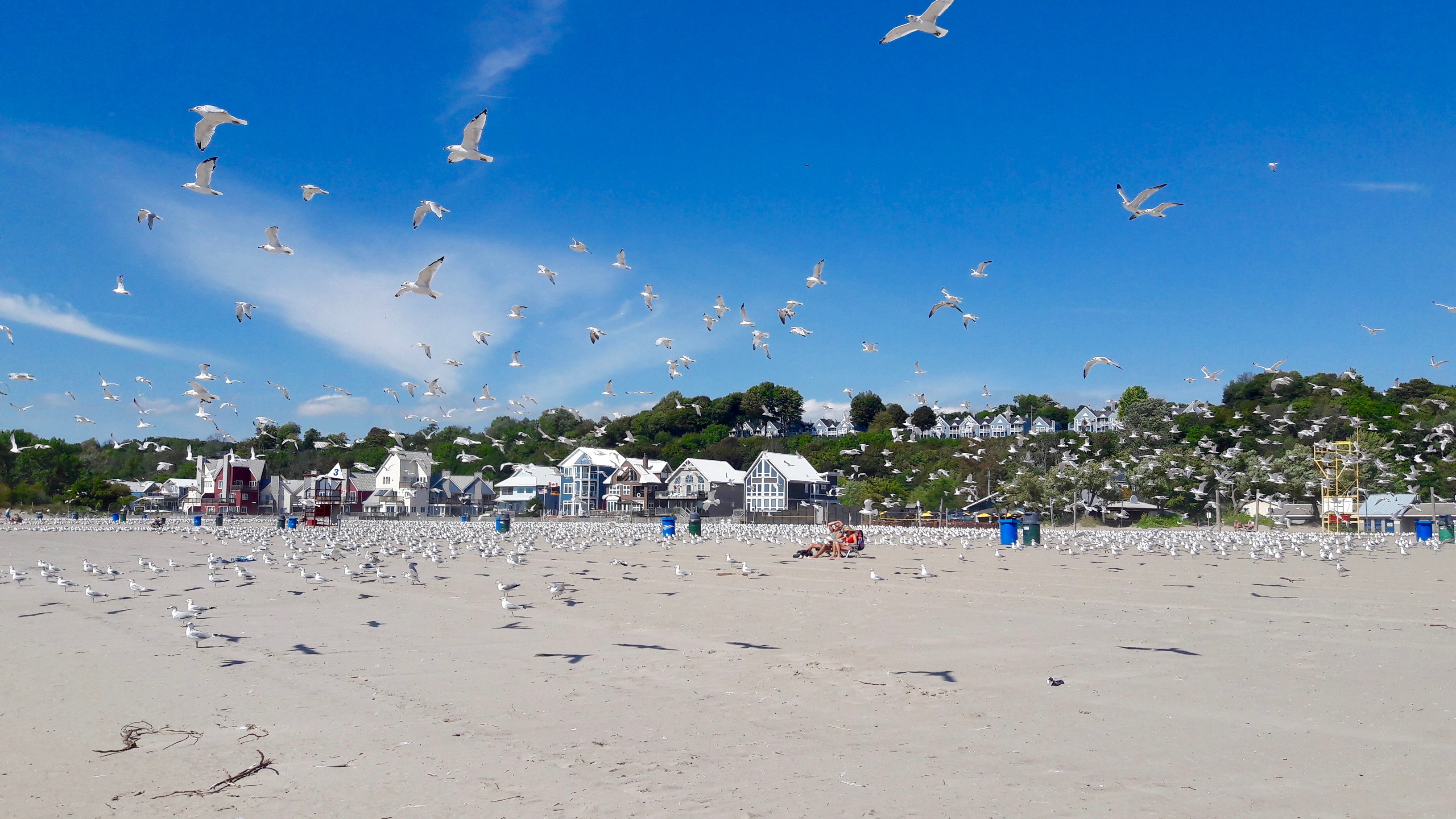Photo number 12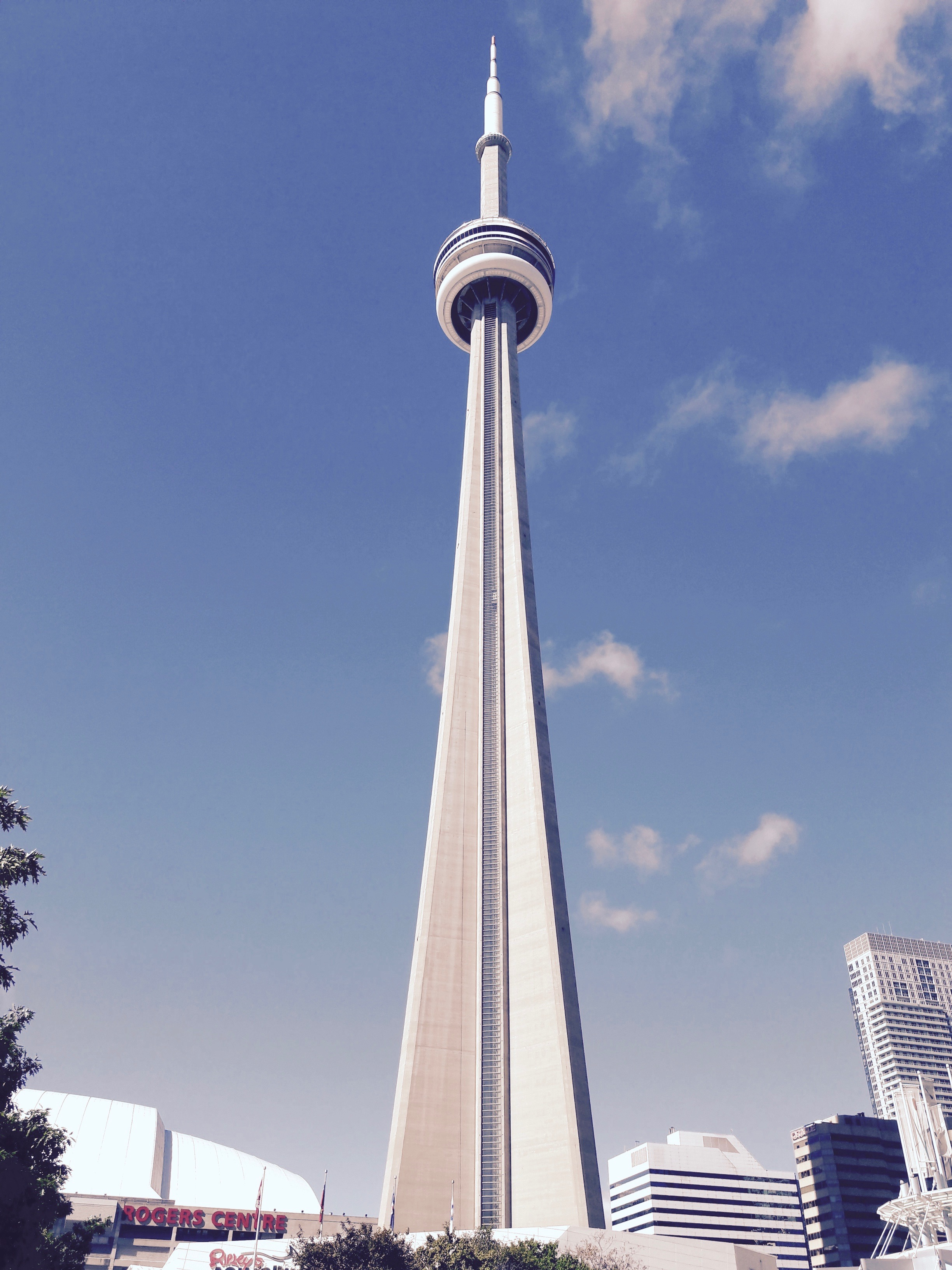Photo number 13Photo number 14Photo number 15Photo number 16Photo number 17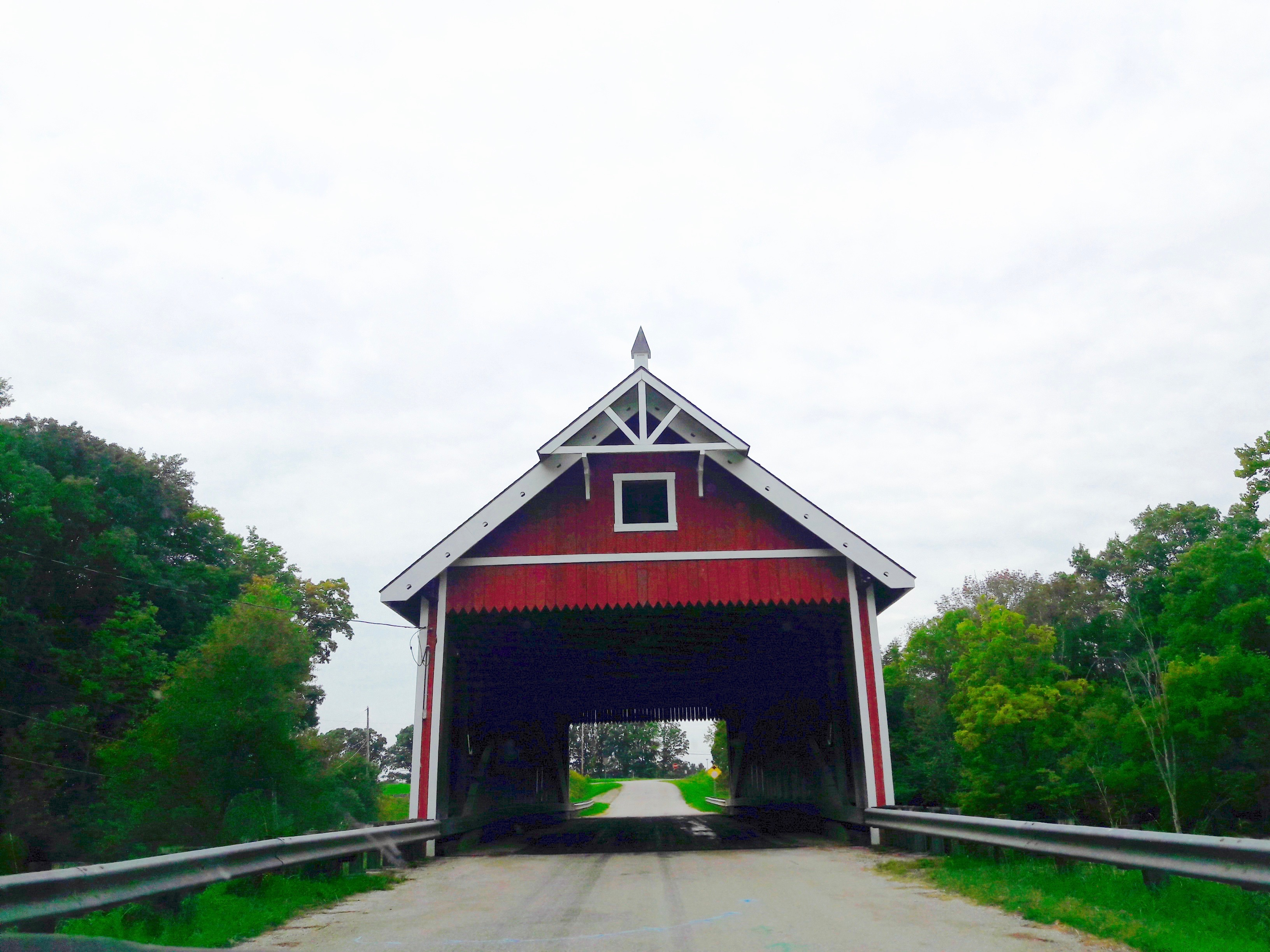Photo number 18Photo number 19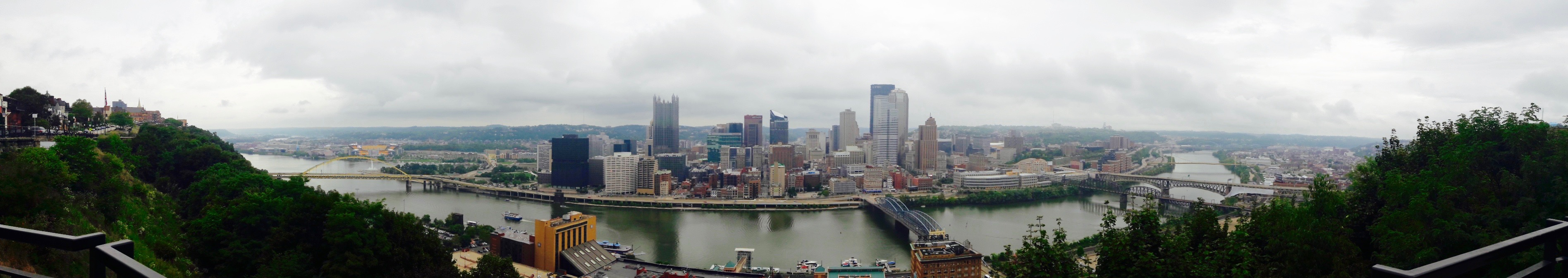Photo number 20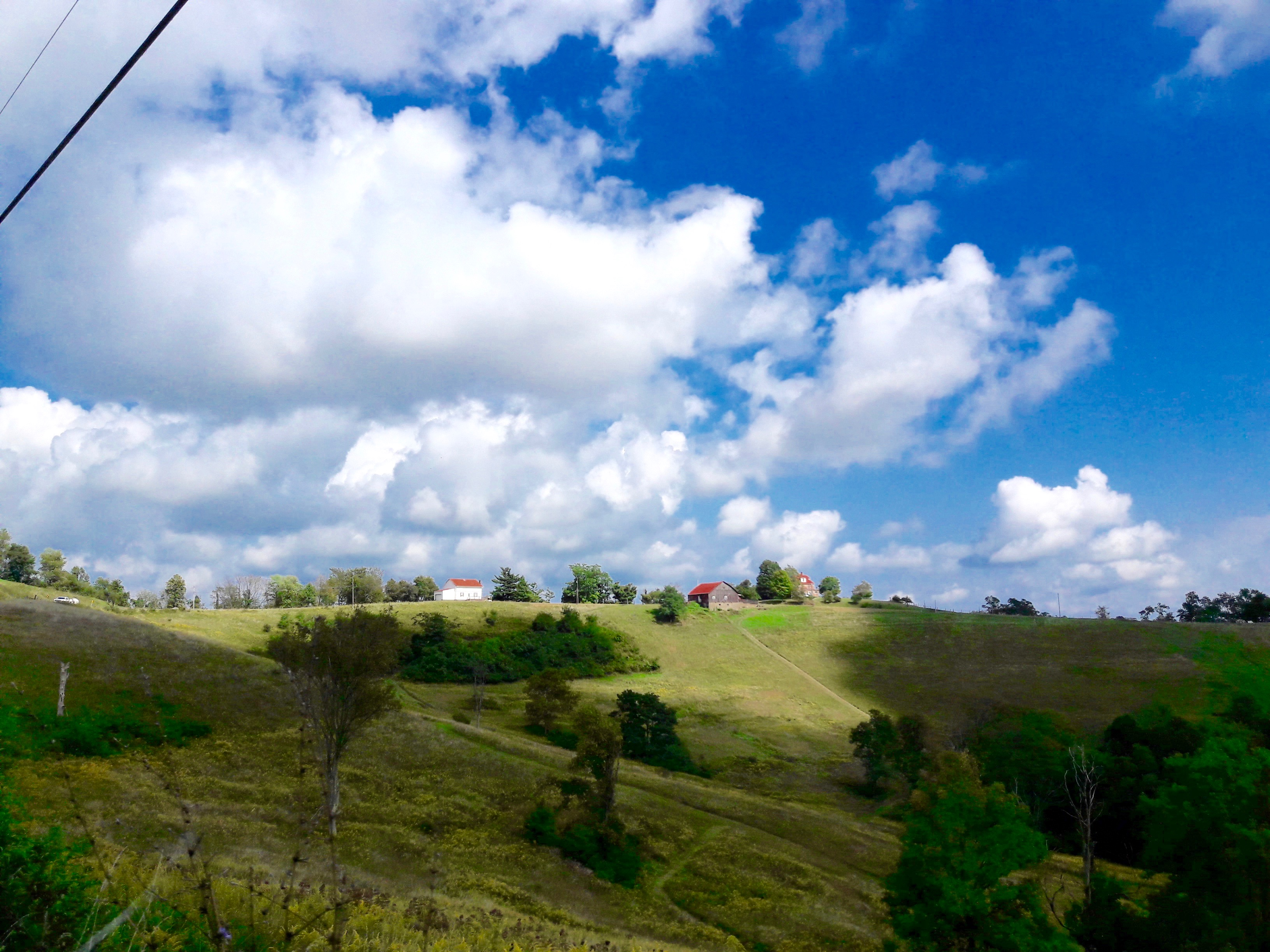Photo number 21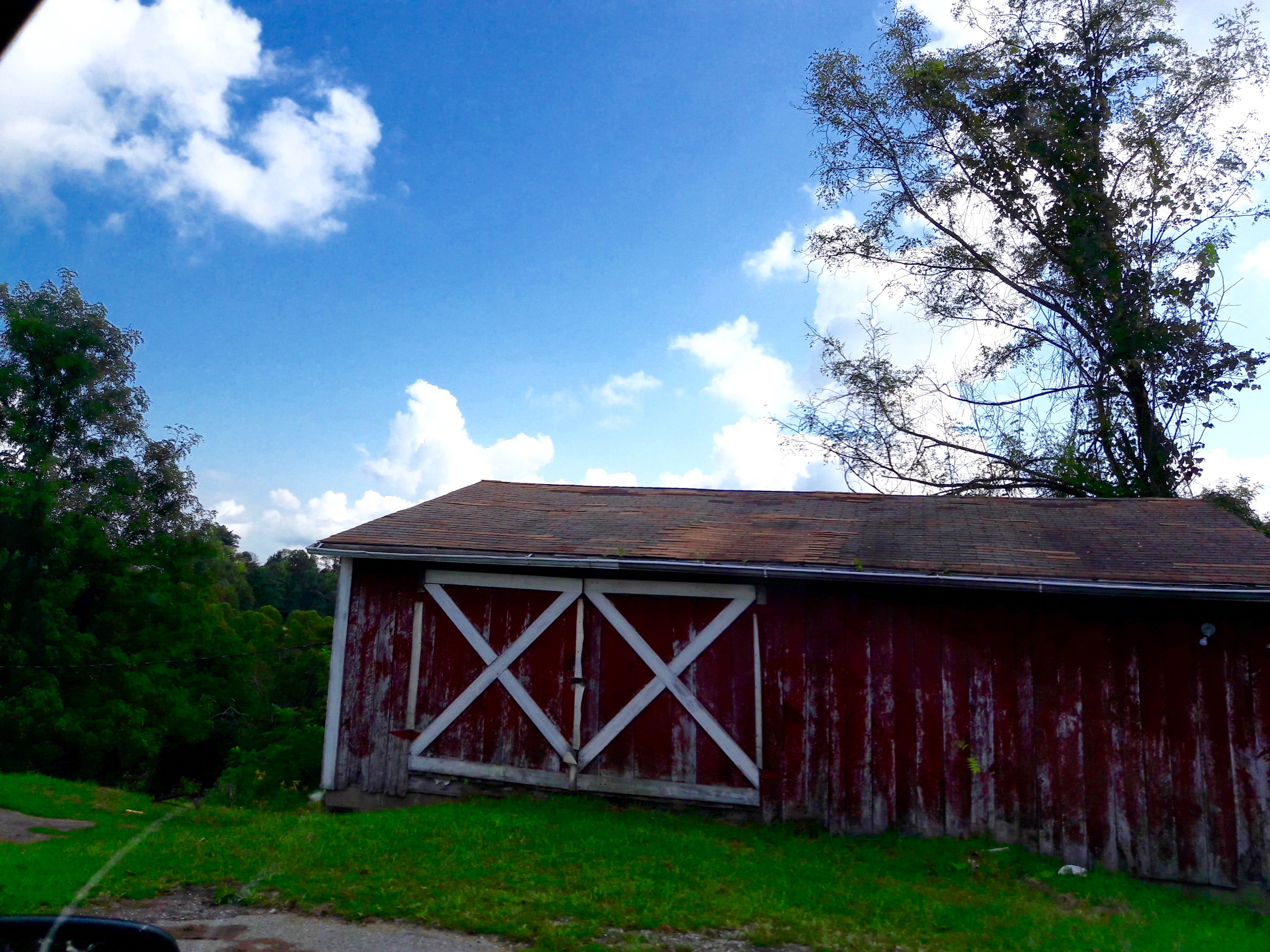Photo number 22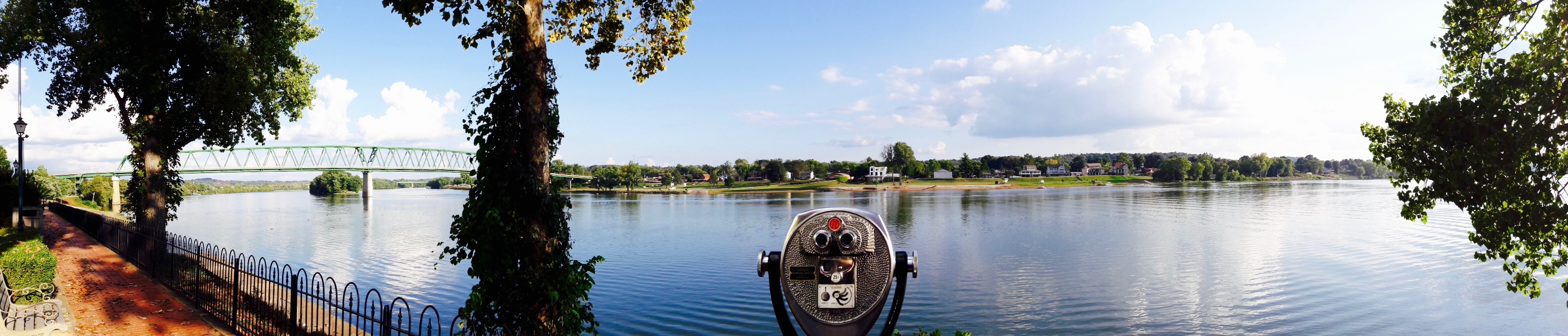Photo number 23Photo number 24Photo number 25Photo number 26Photo number 27Photo number 28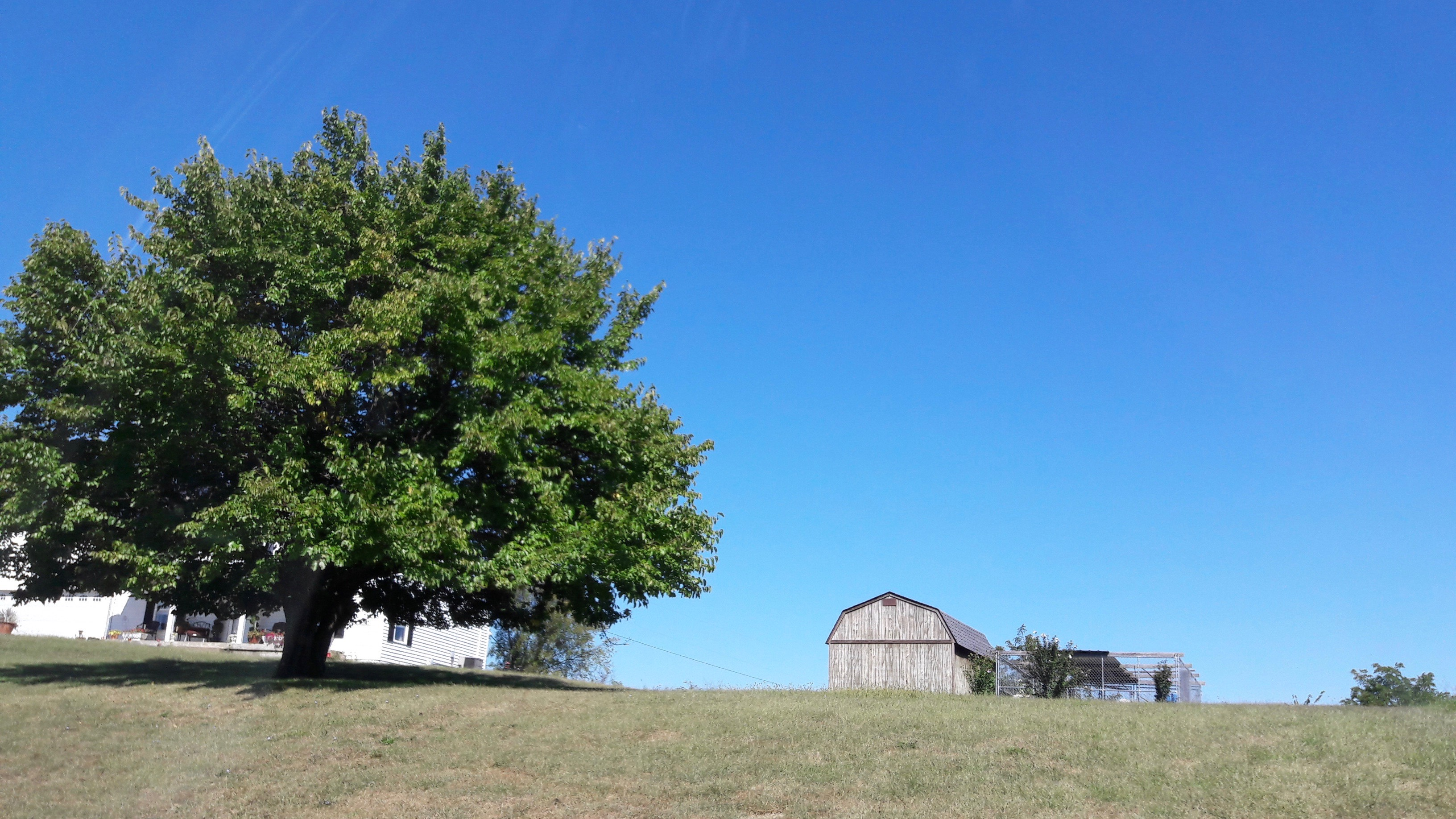Photo number 29Photo number 30Photo number 31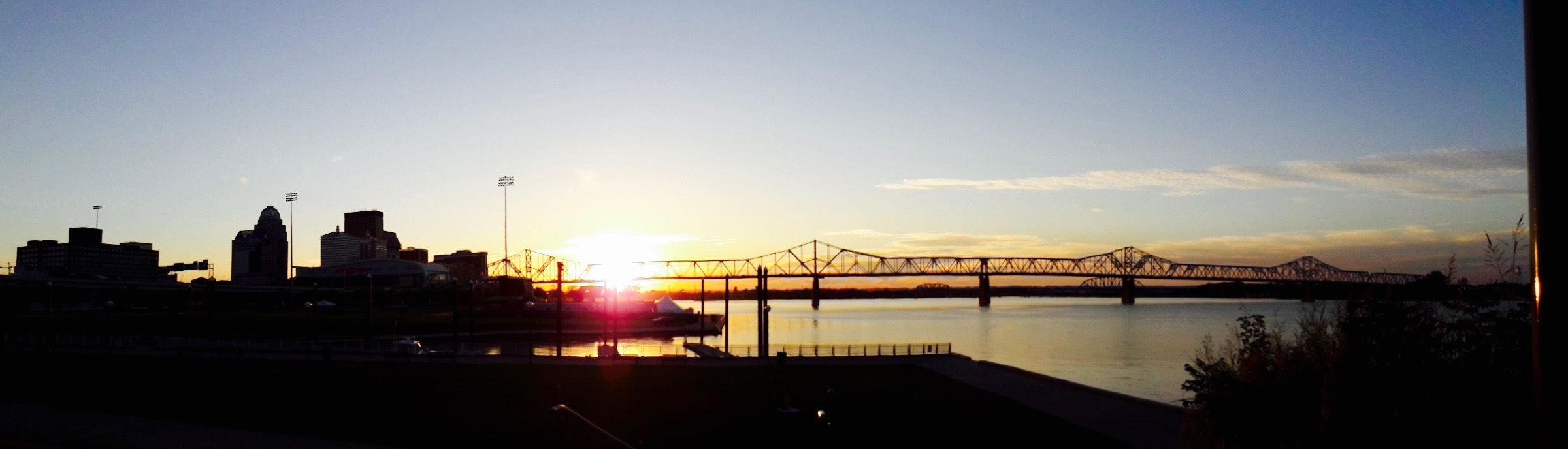Photo number 32Photo number 33Photo number 34Photo number 35